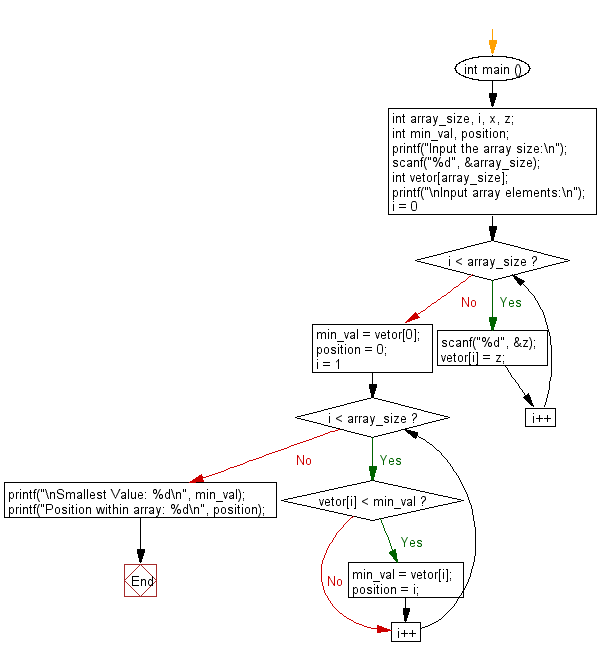﻿ C Program: Lowest value and position within an array - w3resource

# C Exercises: Lowest value and position within an array

## C Basic Declarations and Expressions: Exercise-130 with Solution

Write a C program to create an array of length n and fill the array elements with integer values. Find the smallest value and its position in the array.

Sample Solution:

C Code:

``````#include <stdio.h>
int main ()
{
int array_size, i, x, z;
int min_val, position;

// Prompt the user for the array size
printf("Input the array size:\n");
scanf("%d", &array_size);

// Declare an array of integers with the specified size
int vetor[array_size];

// Prompt the user to input array elements
printf("\nInput array elements:\n");
for (i = 0; i < array_size; i++)
{
scanf("%d", &z);
vetor[i] = z; // Store the input value in the array
}

// Initialize min_val and position with the first element of the array
min_val = vetor;
position = 0;

// Loop to find the smallest value and its position within the array
for (i = 1; i < array_size; i++)
{
if (vetor[i] < min_val)
{
min_val = vetor[i]; // Update the minimum value
position = i; // Update the position of the minimum value
}
}

// Print the smallest value and its position
printf("\nSmallest Value: %d\n", min_val);
printf("Position within array: %d\n", position);

return 0; // End of program
}
``````

Sample Output:

```Input the array size:
5

Input array elements:
35
17
-5
45
36

Smallest Value: -5
Position within array: 2
```

Flowchart:C programming Code Editor:

What is the difficulty level of this exercise?

Test your Programming skills with w3resource's quiz.

﻿